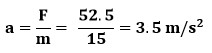## Frictional Force (Friction)

Learn how an object would accelerate after force is applied and a frictional force is created. See how to use the force of friction equation.

Friction force (Ff) is a force caused because all surfaces are rough at the microscopic level.  Therefore a frictional force is created opposite to the direction motion will occur.  Static frictional force is equal to the force applied until a maximum amount and an object starts moving.  Once moving the object has what is called moving or kinetic friction.

• Static Friction: frictional force caused by an applied force at rest (before motion).
• Kinetic Friction: frictional force when an object is in motion.A General Rule: Kinetic (moving or sliding) friction is less than static (standing) friction.

Because moving surfaces are bouncing off each other with little less surface area interaction.### Frictional Force Equation

• Ff=μFN

The Variables and Units:

• Ff : force of friction (N)
• µ : coefficient of friction (no unit)
• FN : normal force (N)

### Frictional Force on a Horizontal (Flat) Surface:

• FN : represents the normal force which the ground pushes up against and equal to weight
• If given mass, solve for Fw (Fw=mg) to know what FN will be equal to.### Different Coefficient of Friction (µ) Variables

• µs represents the coefficient of static friction
• µk represents the coefficient of kinetic sliding friction
• µr represents the coefficient of kinetic rolling friction (wheel rolling)

### More Facts About Coefficient of Friction (µ)

• The bigger the coefficient of friction (µ) the more friction
• Use the same equation but with the right situational value
• µk almost always less than µs

Note:

• The force of static friction is always equal to the force applied until the object begins sliding.
• When you solve for the force of static friction it tells you maximum amount of force before an object would move.### Net Force Equals Zero When in Constant Motion

An object in constant motion has a net force is zero and with no acceleration.

This means the applied forces equals kinetic frictional force.

If applied force was ever more than the kinetic friction

• The object will have a net force
• Net force causes acceleration, not just motion### Example Problems:

It takes 45N of force horizontally to move a wood block with a constant velocity across a desk.

1. What is the applied force?

45 N

Constant velocity means no net force or acceleration. See the picture below2. What is the force of friction?

45 N3. What is the acceleration?

0 m/s24. What is the net force?

0 N5. What force is the minimum you have to apply horizontally to get a 1.5 kg block of wood at rest to move on a wood surface?Minimum to move so up till its still not moving: use static friction

Find normal force By finding weight.

Note: I used 10 m/s2 for the acceleration due to gravity in this example

FN = -FW

Fw = mg

Fw = (1.5)(10)= 15N down

FN = 15N up

Find the force of static friction (Wood on Wood)

Ff = µFN

Ff = (0.25)(15) = 3.75N

6. How much force would it take to keep the 1.5 kg block of wood moving at a constant rate after its moving?

FN = 15N up

Ff = µFN

Ff = (0.20)(15) = 3.0 N

7a. Would a 15kg brick on a wood floor accelerate when 120N of force is applied?

FN = -FW

Fw = mg

Fw = (15)(10)= 150N down

FN = 150N up

Ff = µFN

Ff = (0.60)(150) = 90N

Yes, it would 120N greater than 90N

7b. What would its acceleration be?

We can’t use the Ff from before since the object is moving and now requires the coefficient of kinetic friction

Ff = µFN

Ff = (0.45)(150) = 67.5N

Fnet = 120 – 67.5 = 52.5N forward

F = ma8. If you lift the side of a desk creating an incline until the moment an object first starts moving. What would happen next keeping that exact same angle and why?

A. The block would stop

B. The block would move at a constant rate down the incline

C. The block would accelerate down the incline

Why?

( Choice C ) The block would accelerate down the incline

Because: Kinetic friction is less than static friction.  So as soon as there is motion the force of kinetic friction drops below the force of static friction.  While the force downhill that caused the motion stays the same.  Therefore there is a net force which causes acceleration and not just motion

(See the animation of the forces interacting below)Notice how frictional force uphill and parallel force downhill increase with the incline until motion occurs.  Because of motion, static friction changes to a lesser kinetic friction.  Therefore the force uphill (friction) is less than the force downhill.  At that moment the net force is downhill.  So the net force downhill creates an acceleration.Friction Quiz

1 / 7

What type of friction do you have while an object is at rest?

2 / 7

What is the magnitude of frictional force when 162 N of force is required to keep an object in constant motion?

3 / 7

What is the magnitude of net force when 162 N of force is required to keep an object in constant motion?

4 / 7

Which is almost always greater

5 / 7

You use a spring scale to measure the amount of force required to first start an object moving.  You would use this value to determine the coefficient of ___________ friction.

Force applied equals the magnitude of force of friction in constant motion.  If you find the force of friction (Ff), you know the Force applied (FA)

6 / 7What is the force required to keep a 0.5 kg brick in constant motion on a wood surface?

7 / 7What is the minimum force required to start a 0.5 kg brick moving on a wood surface?